# Excel WBS and Gantt Chart - XLGantt How to #4 – Keeping track the progress of the project

You can use "%work complete(progress)" to keep track of the progress of the project. Let's look at how XLGantt(Excel Gantt) manages and tracks the progress of the project.

To track the "%work complete" of individual tasks, see "XLGantt(Excel Gantt) How to #2 – Input task, Update schedule"

### 1. Concept of "%work complete" to track project progress.

To track the overall progress of a project, we use the following two indicators a lot.

#### 1) Planned %work complete

Planned %work complete to the current date. Calculate with the following formula.

#### 2)  %Work complete

The actual %work complete to the current date. Calculate with the following formula.

* In addition, the above two indicators can be used to calculate the achievement rate.

It is possible to quickly determine whether a goal has been achieved(based on the current date). Calculate with the following formula.

= %Work complete/Planned %work complete

### 2. How to track %work complete in XLGantt

XLGantt automatically calculates the above indicators and can be found on the Schedule sheet and Progress sheet.

#### 1) First, let's find out how to calculate the workload which is the basis of %work complete calculation.

* The workload is based on Man/Day. Man/Day is the number of people assigned during a particular period. If 5 Man/Day, 5 people are assigned for 1 day, or 1 person is assigned for 5 days.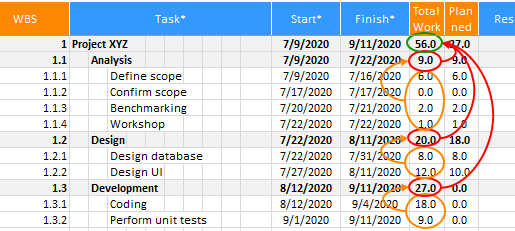The actual workload is calculated only when the "%work complete" is entered.

- The actual workload of "Define scope" task is 6 because the %work complete was entered as 100%.
- The actual workload of "Design Database" task is 8 because the %work complete was entered as 100%.
- The actual workload of "Design UI" is 12*80% = 9.6 since only 80% of total work was completed.
When summed up, the actual workload is 6+8+9.6 = 23.6.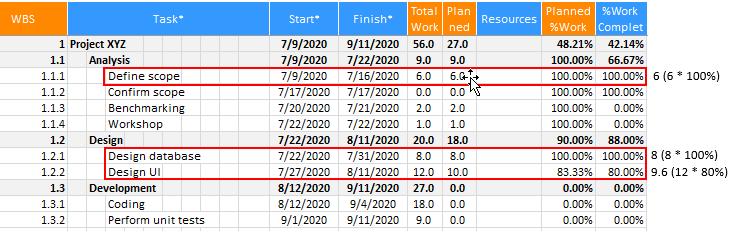#### 2) %Work complete calculation

The planned %work complete and actual %work complete are calculated in the following manner and can be found in the Schedule sheet and Progress sheet.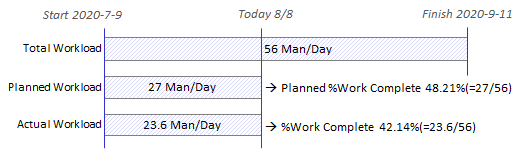#### 3) Display Progress

The upper part of the Schedule sheet

After entering tasks, click "Update Schedule" on the ribbon. The planned progress rate and the actual progress rate are calculated and displayed at the upper part of the sheet.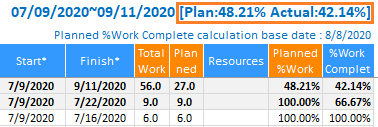Progress sheet

After entering tasks, click "Update Schedule" on the ribbon. Indicators are automatically calculated and displayed on the Progress sheet as shown below.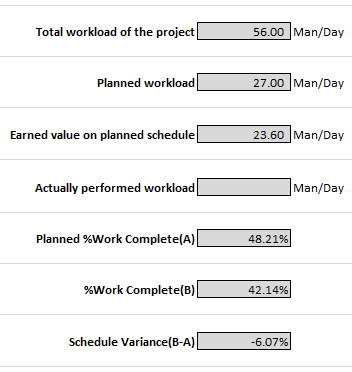* Tip – What if you want to see progress rate in the future or past?

There may be times when you need to find a "planned progress" at a certain point in project practice. For example, today's Wednesday, and you're looking for the "planned progress" on the coming Friday.

Set the date you want in the ribbon menu> XLGantt > Options> General> "Calculation Base Date of Planned %Work Complete".
(If you select "Today", it is always calculated based on the current day).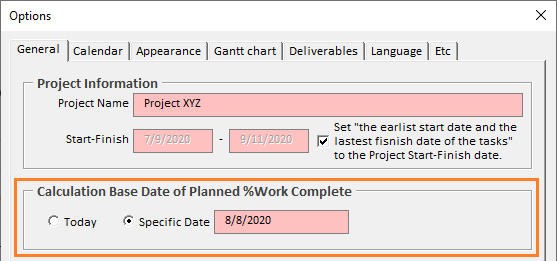[ XLGantt(Excel Gantt) How to ]
Scroll to Top